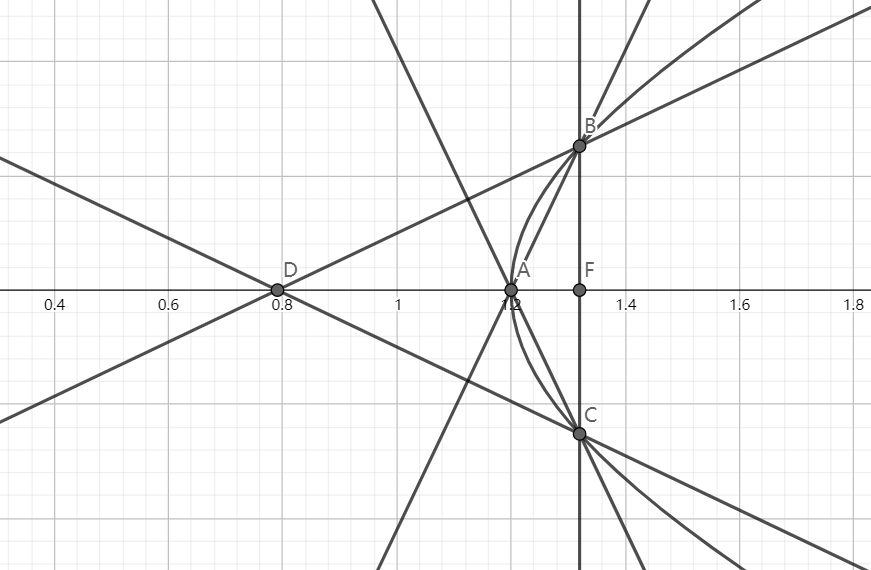# SAT1000 - P767

Geometry Level pendingAs shown above, the hyperbola: $\dfrac{x^2}{a^2}-\dfrac{y^2}{b^2}=1\ (a>0,b>0)$ has right focus point $F$, right vertex $A$.

Line $l_1$ passes through $F$ and it intersects with the hyperbola at point $B,C$, $l_2$ passes through $B$ and $l_2 \perp AC$, $l_3$ passes through $C$ and $l_2 \perp AB$, $l_2, l_3$ intersects at point $D$.

If the distance from $D$ to line $l_1$ is less than $a+\sqrt{a^2+b^2}$, what is the range of the slope of the hyperbola's asymptotes?

Have a look at my problem set: SAT 1000 problems

×